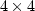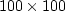Next: Method of random directions Up: Model fitting by least Previous: Unknown input: deconvolution with

# ITERATIVE METHODS

The solution time for simultaneous linear equations grows cubically with the number of unknowns. There are three regimes for solution; which one is applicable depends on the number of unknowns n. For n three or less, we use analytical methods. We also sometimes use analytical methods on matrices of sizeif the matrix contains many zeros. For n<500 we use exact numerical methods such as Gauss reduction. A 1988 vintage workstation solves asystem in a minute, but asystem requires a week. At around n=500, exact numerical methods must be abandoned and iterative methods must be used.

An example of a geophysical problem with n>1000 is a missing seismogram. Deciding how to handle a missing seismogram may at first seem like a question of missing data, not excess numbers of model points. In fitting wave-field data to a consistent model, however, the missing data is seen to be just more unknowns. In real life we generally have not one missing seismogram, but many. Theory in 2-D requires that seismograms be collected along an infinite line. Since any data-collection activity has a start and an end, however, practical analysis must choose between falsely asserting zero data values where data was not collected, or implicitly determining values for unrecorded data at the ends of a survey.

A numerical technique known as the conjugate-gradient method" (CG) works well for all values of n and is our subject here. As with most simultaneous equation solvers, an exact answer (assuming exact arithmetic) is attained in a finite number of steps. And if n is too large to allow n3 computations, the CG method can be interrupted at any stage, the partial result often proving useful. Whether or not a partial result actually is useful is the subject of much research; naturally, the results vary from one application to the next.

 The simple form of the CG algorithm covered here is a sequence of steps. In each step the minimum is found in the plane given by two vectors: the gradient vector and the vector of the previous step.Next: Method of random directions Up: Model fitting by least Previous: Unknown input: deconvolution with
Stanford Exploration Project
10/21/1998